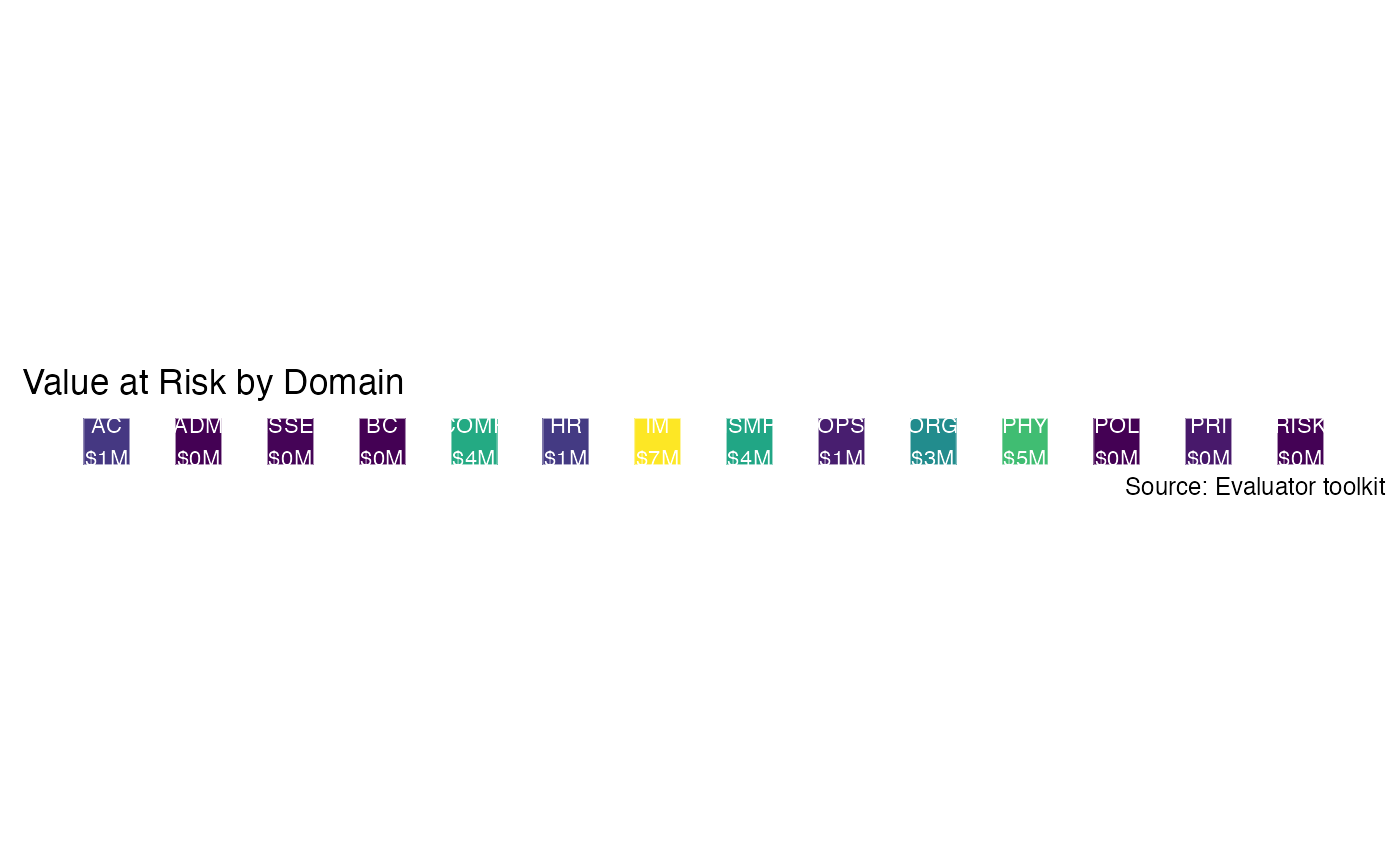Given a domain_summary and a list of all domains, generate a heatmap colored by the 95% VaR. This plot displays the domains in which aggregate risk is greater than others.

generate_heatmap(domain_summary)

## Arguments

domain_summary Simulations summarized at a domain level via summarize_domains.

## Value

A ggplot object.

Other result graphs: exposure_histogram(), generate_event_outcomes_plot(), generate_scatterplot-deprecated, loss_exceedance_curve(), loss_scatterplot()
data(mc_domain_summary)
generate_heatmap(mc_domain_summary)